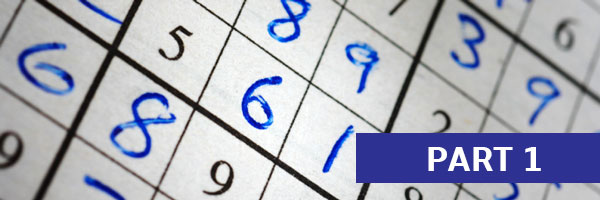# How to Solve Sudoku Puzzles – A Complete Walkthrough, Part 1First, Look for “Low-Hanging Fruit”

Sudoku involves filling empty spaces with the number 1-9, without repeating any numbers within each row, column or square. This means that the entire puzzle should be able to be solved without guessing – that means you can use deductive reasoning, based solely on which numbers are already in place within each row, column or square, to figure out which numbers should go in which empty spaces.

One of the best ways to get started with solving a Sudoku puzzle is to look for “low-hanging fruit” – the rows, columns or squares that already have the most numbers filled in.

This will make it easier (most of the time) for you to figure out which numbers go in the remaining empty spaces.

For example, in this puzzle to above, the column and row that are outlined in blue, and the squares outlined in green (in the lower right), all have only three empty spaces.

That means that these sections of the Sudoku puzzle are a good place to start.

Let’s start by looking at the center square in the bottom row of the grid, shown here (in the figure below) outlined in green.

Use Process of Elimination

We know that there are three empty spaces in this square, which must contain the missing numbers of 5, 6 and 7. If we look to nearby squares for other instances of 6 and 7, we might be able to eliminate possibilities and see which number should go in which space.

Fortunately, if we look to the top row of the grid, we see a 7 (circled in red) and in the lower right corner of the grid (bottom row) we see a 6 (circled in red).

This means that the empty space (circled in blue) in the bottom row cannot contain 7 or 6, and must contain number 5.

Now let’s look to the other “low-hanging fruit” square, in the lower-right corner, shown in the figure to the left, outlined in green.

This square also has only three empty spaces. By checking to see which numbers are already in place (1, 2, 4, 6, 8 and 9), we know that the missing numbers are 3, 5 and 7.

If we look above, we can see a 5 and 7 in the central row of the square (circled in red), so that means that the top center space must contain a 3 (in blue). Also, if we look to the left, we can see 5 (circled in red), which means that the upper left space of the square cannot contain 5, so that means the 5 has to go in the left-center space (marked in blue) and the last remaining empty space has to be a 7. This is how we can use simple process of elimination to figure out which numbers go in which spaces, starting with the “low-hanging fruit” that is given to us by the Sudoku grid.

Please read part 2 of this series on how to solve Sudoku puzzles, using this actual game as an example. (Coming soon!)